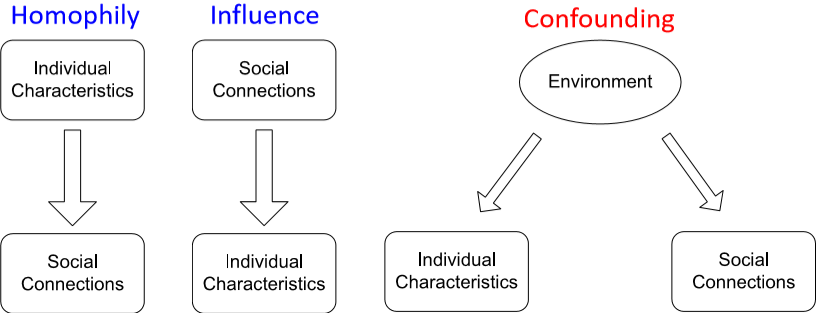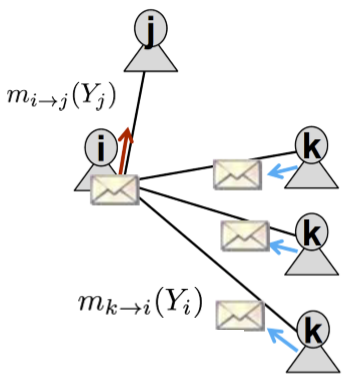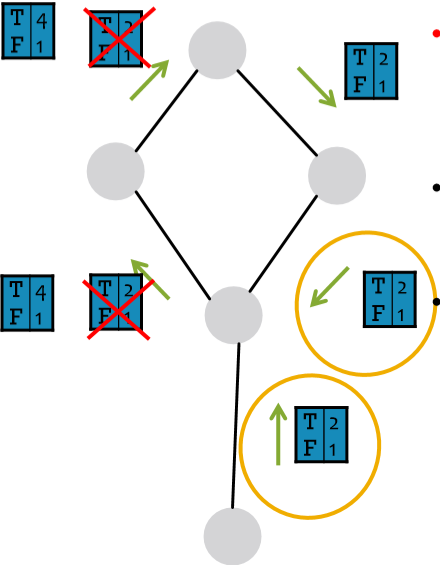# 【图机器学习】cs224w Lecture 6 - 消息传递 及 节点分类

• relational classification
• iterative classification
• belief propagation

exact inference vs. approximate inference

## Node Classification• homophily：“物以类聚，人以群分”，有相同性质的节点间可能存在更密切的联系
• influence：“近朱者赤，近墨者黑”，一个个体有可能会受其它个体的影响而具有某种性质
• confounding：大环境可能会对个体性质和个体间的联系产生影响

Collective classification 大体分为三步：

1. local classifier：就像一般的分类任务一样，只依赖节点自己的特征信息而不牵扯任何网络信息
2. relational classifier：考虑邻接点的标签和特征
3. collective inference：迭代地将 relational classifier 应用在每个节点上，相当于拓展了节点的“感知野”

### Probabilistic Relational Classifier

$P(Y_i=c)=\frac1{\sum_{(i,j)\in E}W_{ij}}\sum_{(i,j)\in E}W_{ij}P(Y_j=c)$

### Iterative Classification

slide 里给了一个用 word-bag 作为特征的网页分类的例子，感兴趣可以去看看。

• reviewer: $F(u)=\frac{\sum\limits_{(u,p)\in Out(u)}R(u,p)}{|Out(u)|}$
• review: $R(u,p)=\frac1{\gamma_1+\gamma_2}(\gamma_1F(u)+\gamma_2(1-\frac{|score(u,p)-G(p)|}2))$
• 网页: $G(p)=\frac{\sum\limits_{(u,p)\in In(p)}R(u,p)\cdot score(u,p)}{|In(p)|}$

Michele 的回答是这样做只会让这个人的评分趋近 $0.5$ 而不会像那种给好网页评负分的真正的 fake reviewer 那样得分趋近 $0$

### Belief Propagation• label-label potential matrix $\psi(Y_i,Y_j)$：表示节点 $i$ 是类别 $Y_i$ 的条件下，其邻接节点 $j$ 为类别 $Y_j$ 的概率
• prior belief $\phi_i(Y_i)$：表示节点 $i$ 为类别 $Y_i$ 的先验概率
• $m_{i\rightarrow j}(Y_j)$：节点 $i$ 在多大程度上认为其邻接节点 $j$ 是类别 $Y_j$ (不知道这里能不能算作是一个概率)

$m_{i\rightarrow j}(Y_j)=\alpha\sum\limits_{Y_i\in L}\psi(Y_i,Y_j)\phi_i(Y_i)\prod_{k\in N_i\text{\textbackslash}j}m_{k\rightarrow i}(Y_i)$

$b_i(Y_i)=\alpha\phi_i(Y_i)\prod_{j\in N_i}m_{j\rightarrow i}(Y_i)$1. 这里的 weak correlation 不太明白是什么意思。弱相关能解环？还是说这里的 correlation 就是 link 的意思，然后 weak 代表传播的概率在这里很小？ ↩︎

posted @ 2020-04-09 14:43  WineChocolate  阅读(794)  评论(3编辑  收藏  举报